Home / Blog / Artificial Intelligence / Activation Functions

# Activation Functions

• July 13, 2023
• 11321
• 23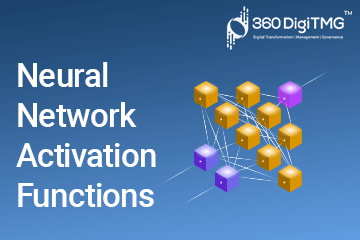### Meet the Author : Mr. Bharani Kumar

Bharani Kumar Depuru is a well known IT personality from Hyderabad. He is the Founder and Director of Innodatatics Pvt Ltd and 360DigiTMG. Bharani Kumar is an IIT and ISB alumni with more than 17 years of experience, he held prominent positions in the IT elites like HSBC, ITC Infotech, Infosys, and Deloitte. He is a prevalent IT consultant specializing in Industrial Revolution 4.0 implementation, Data Analytics practice setup, Artificial Intelligence, Big Data Analytics, Industrial IoT, Business Intelligence and Business Management. Bharani Kumar is also the chief trainer at 360DigiTMG with more than Ten years of experience and has been making the IT transition journey easy for his students. 360DigiTMG is at the forefront of delivering quality education, thereby bridging the gap between academia and industry.

Table of Content

The activation function is the most important aspect of deep learning. Knowing the outcome from the inputs is helpful.

They have a significant influence on whether neurons are activated or deactivated. For complicated neural networks, the input layer is transformed nonlinearly to provide precise outputs. The supplied data may be normalised using it.

Integration and activation are the two fundamental components of a neuron.

The weighted average of the input is provided by the integration component, and the activation function uses this number to produce an output.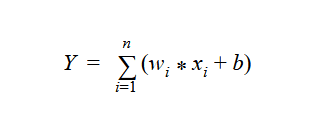## Why Activation Function?

Without an activation function, a neural network will become a linear regression model. But introducing the activation function the neural network will perform a non-linear transformation to the input and will be suitable to solve problems like image classification, sentence prediction, or langue translation.

There are multiple types of activation

• Linear:

A function whose activation is inversely correlated with the input. The weighted total of the input will be the output. The identity function is another term for a linear activation function.

A neural network is identical to a linear regression model if it merely has a linear activation function. It is unable to manage complicated data with variable parameters.

Gradient descent cannot be accomplished with this activation function since its derivative is constant. Because of this, it is not feasible to adjust the weights afterwards.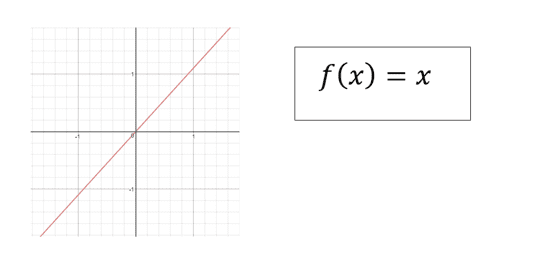• ReLU:

It is called a rectified linear unit, if the value is greater than 0, then it will give away the same value as output. otherwise, it will give 0 as output.

ReLU will help the network to converge quickly. It simply looks like a linear function but it takes care of backpropagation.

However, when the inputs become zero or negative, the gradient of the function becomes zero and hence will not perform the backpropagation operation. This is called "The dying ReLU".

Also, this activation function should be only used in hidden layers of a neural network.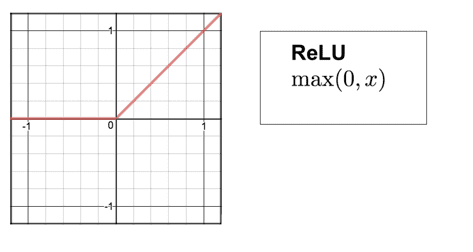• ELU:

Similar in operation to the ReLU, the exponential linear unit also takes the negative value into account. Give the same value as an output if the value is larger than 0, else it gives (ex-1) where is a positive constant integer.

By examining the graph, we can see that ELU gradually gets smooth until its output equals -. ELU is strongly employed as a substitute for ReLU in order to avoid the "The Dying ReLU" problem.

However, there is a drawback to ELU: if the output ranges from (0, ∞), it can burst the activation function for x > 0.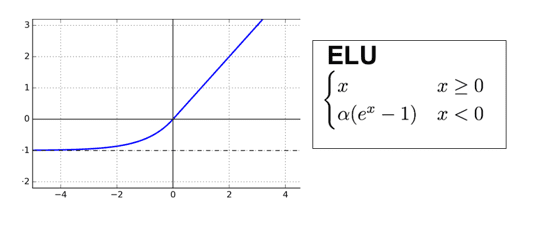• Sigmoid/ Logistic:

A function which takes values as input produces an output within the range of 0 to 1. It is easy to work with as it is continuous, has a fixed range of outputs, and it is differentiable.

The gradient of the sigmoid activation is smooth and can be used as a good classifier. Unlike the linear activation function where the ranges were from (0, ), it has a range (0,1) which will not explode the activation functions. Click here to learn Data Analytics in Bangalore

However, the output values tend to respond less to the changes in inputs giving raise to the vanishing gradient problem. Also, the outputs are not zero centred, it makes the gradient go too far in different directions which are going to make the optimization harder.

The computation process is expensive.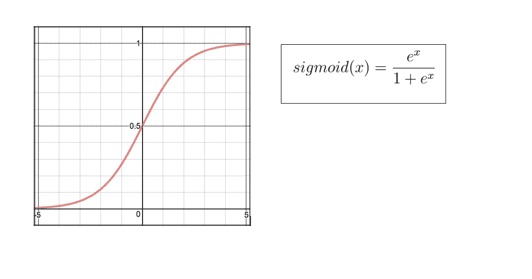• Tanh:

A comparable activation function to the sigmoid is the hyperbolic tangent, although whose output values span from -1 to 1. Tanh is chosen over sigmoid because its output is zero-centered in contrast to sigmoid's.

While Tanh outperforms sigmoid activation functions, it still remains true for the vanishing gradient problem.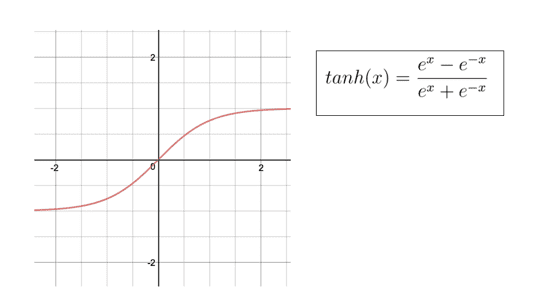• Softmax:

An activation function which will calculate the probabilities of target class overall the target classes. The output of each class is normalized between 0 and 1 and the resulting probability lets know the class of the input. Click here to learn Python in Hyderabad

Sometimes, the names SoftMax and sigmoid will confuse as both the names start with “S” and the values of the outputs are also almost similar(0,1).

One thing to keep in mind about the SoftMax activation function is, it’s been only used in the output layer of the neural network which will solve the multiple class problem.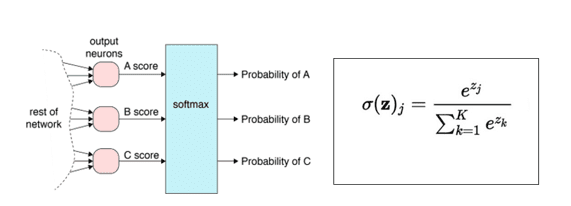• Heaviside step:

The value of this unit step function is 0 for all negative values and 1 for all positive ones. It is a discontinuous function with Oliver Heaviside as its eponym. They are incredibly helpful for investigations involving binary categorization since they provide binary results.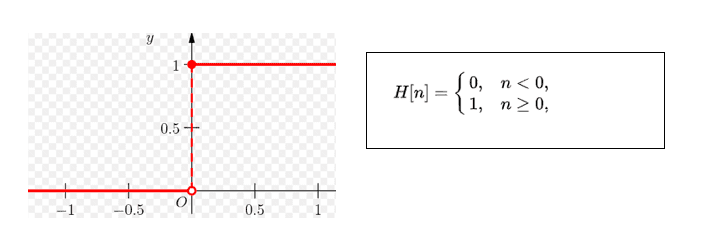• Arctangent:

This activation function is similar to sigmoid and Tanh, it maps the inputs to outputs which range between (-2,2).

Its derivative converges quadratically again 0 for larger values. Whereas, the sigmoid converges exponentially against 0.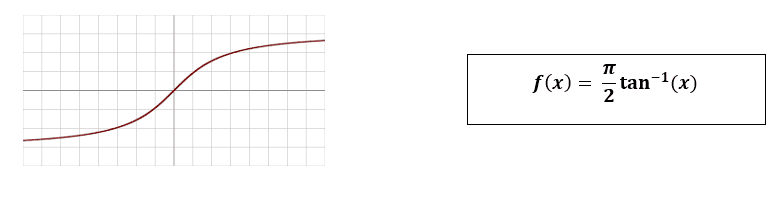• Leaky ReLU:

The ReLU activation function is fairly similar to this one, but Leaky ReLU does take the negative values into account, albeit to a lesser extent.

Through the use of a little negative slope, "The Dying ReLU" problem is being attempted to be fixed. It permits backpropagation even for negative input values thanks to a little positive slope in the negative zone.

It may be utilised to resolve complicated categorization issues because to its linearity. For a few situations, it seems to lag behind the sigmoid and Tanh. Negative values cause it to perform poorly,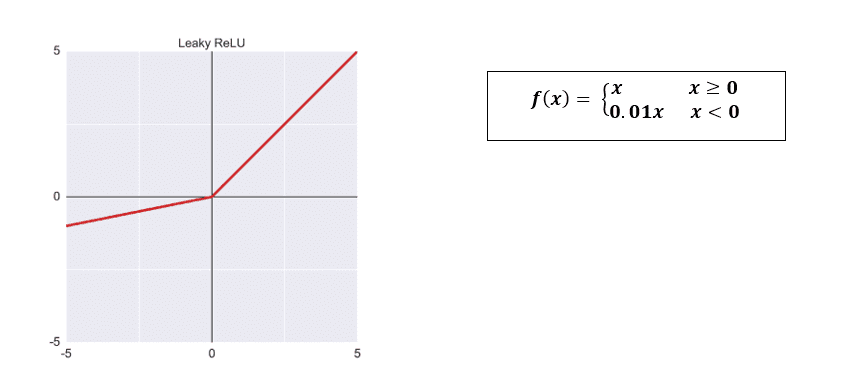• Parametric ReLU:

It is a type of Leaky ReLU where it makes the coefficient of leakage into a parameter.

Leaky Relu gives the negative slope for the negative values, but it is going to behave differently for multiple problems which makes it as one of the disadvantages of this function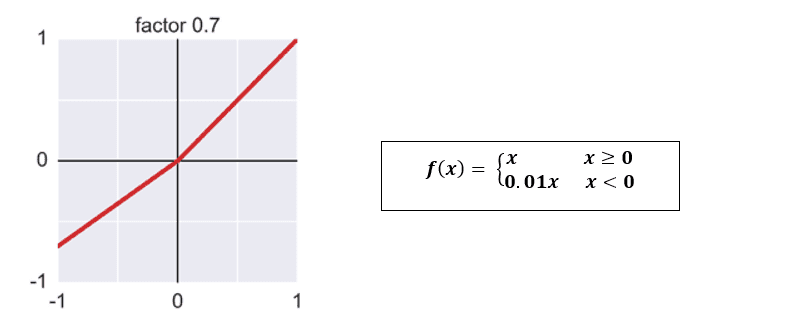• Softplus:

It is a smoothed variant of ReLU; ReLU and Softplus are comparable except that Softplus is smooth and differentiable around 0 whereas ReLU is not.

It was initially introduced in 2001, and by differentiating itself and reducing saturation, it can be utilised to combat "The Dying ReLU" problem.

While the softplus function gives output in the range of (0, ∞), the sigmoid and Tanh functions' outputs have a certain range.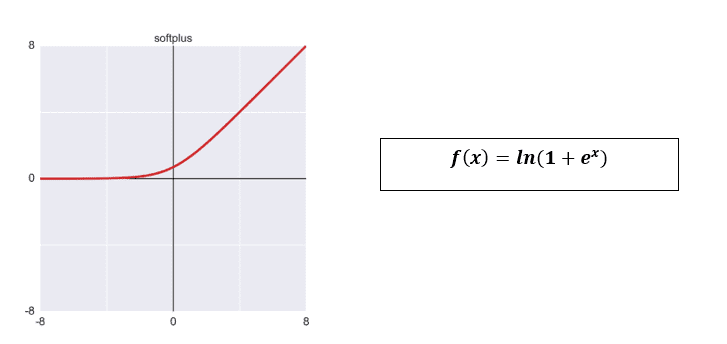• Maxout:

An activation function returns the maximum value among the n values from a linear equation.

It is a combination of ReLU and Leaky ReLU, most of the time it is used along with the drop out technique. Click here to learn Artificial Intelligence in Bangalore

However, the parameters to be learnt by each neuron will be doubled, so it is required to train a lot of parameters.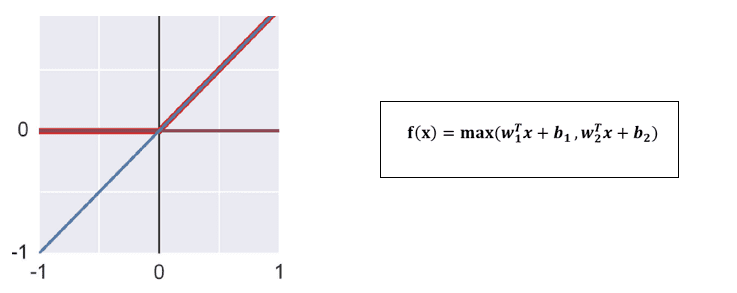• Ramp:

It has a very similar appearance to the sigmoid activation function and translates inputs to outputs throughout the range (0,1), however the ramp will have a steep curve rather than a smooth one. A linear function that has been shortened.• Shifted ReLU:

It is a variation of ReLU which just moves the bend down and left. It has the flexibility to choose horizontal and vertical shifts. Click here to learn Machine Learning in Hyderabad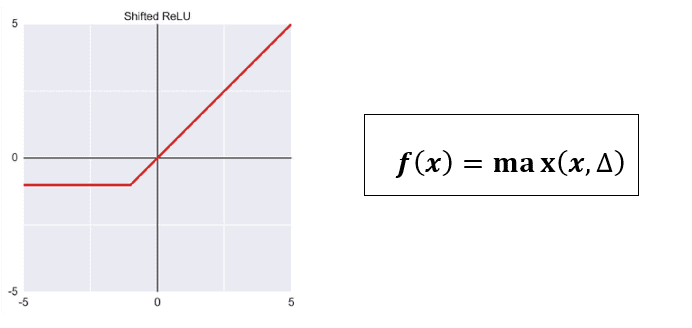• Stair Step:

It outputs x's floor value as the output. In this illustration, the function outputs 0 if the input value is between 0 and just less than 0.2, 0.2 if it is between 0.2 and just less than 0.4, and so on.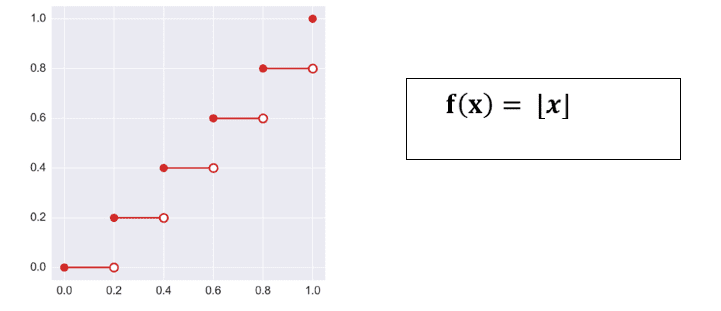• Step:

This is a very basic activation function, a threshold value is decided to give the output. It is used to solve classification and binary class problems.

However,It will not classify the input into other categories.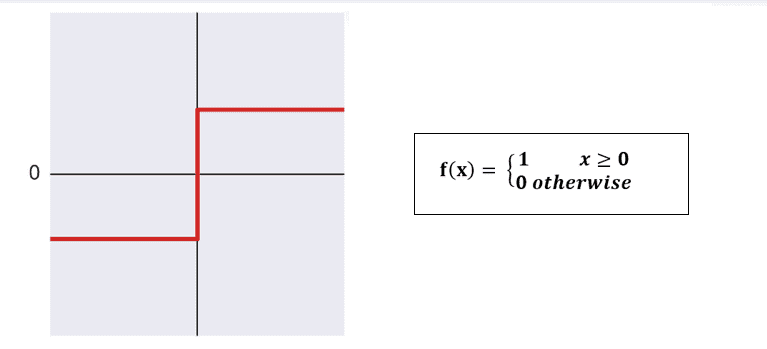• Swish:

It combines the ReLU with the sigmoid. It is a ReLU that has a little, smooth hump immediately to the left of 0 before flattening off.

The Google researchers make the discoveryAccording to them, this activation function outperforms ReLU with a comparable degree of computational efficiency.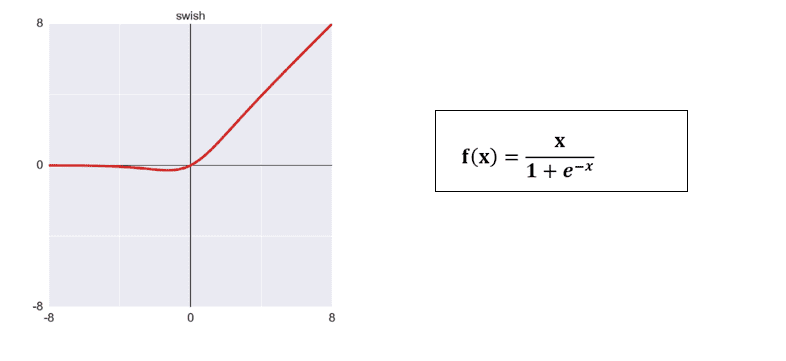### Data Science Training Institutes in Other Locations